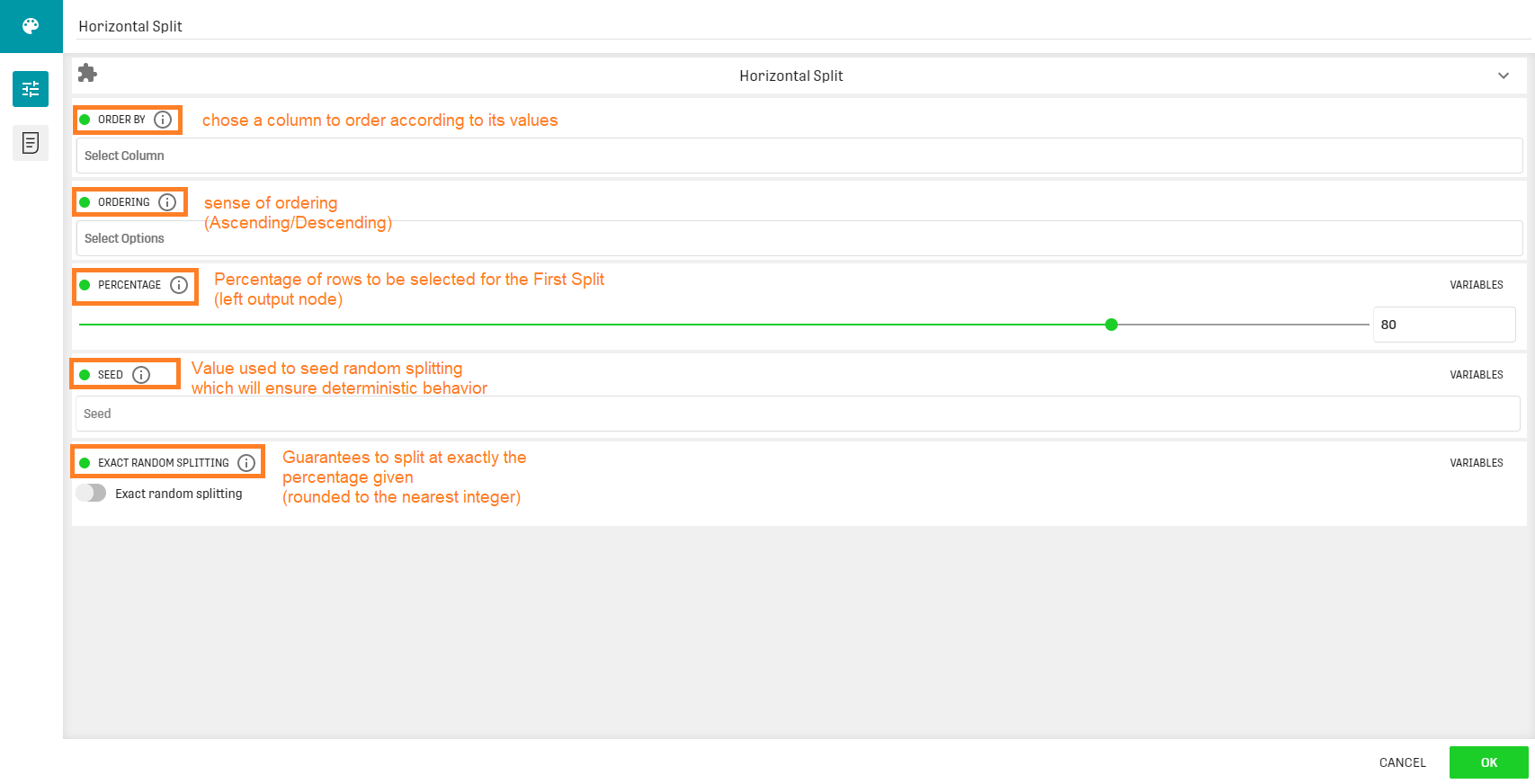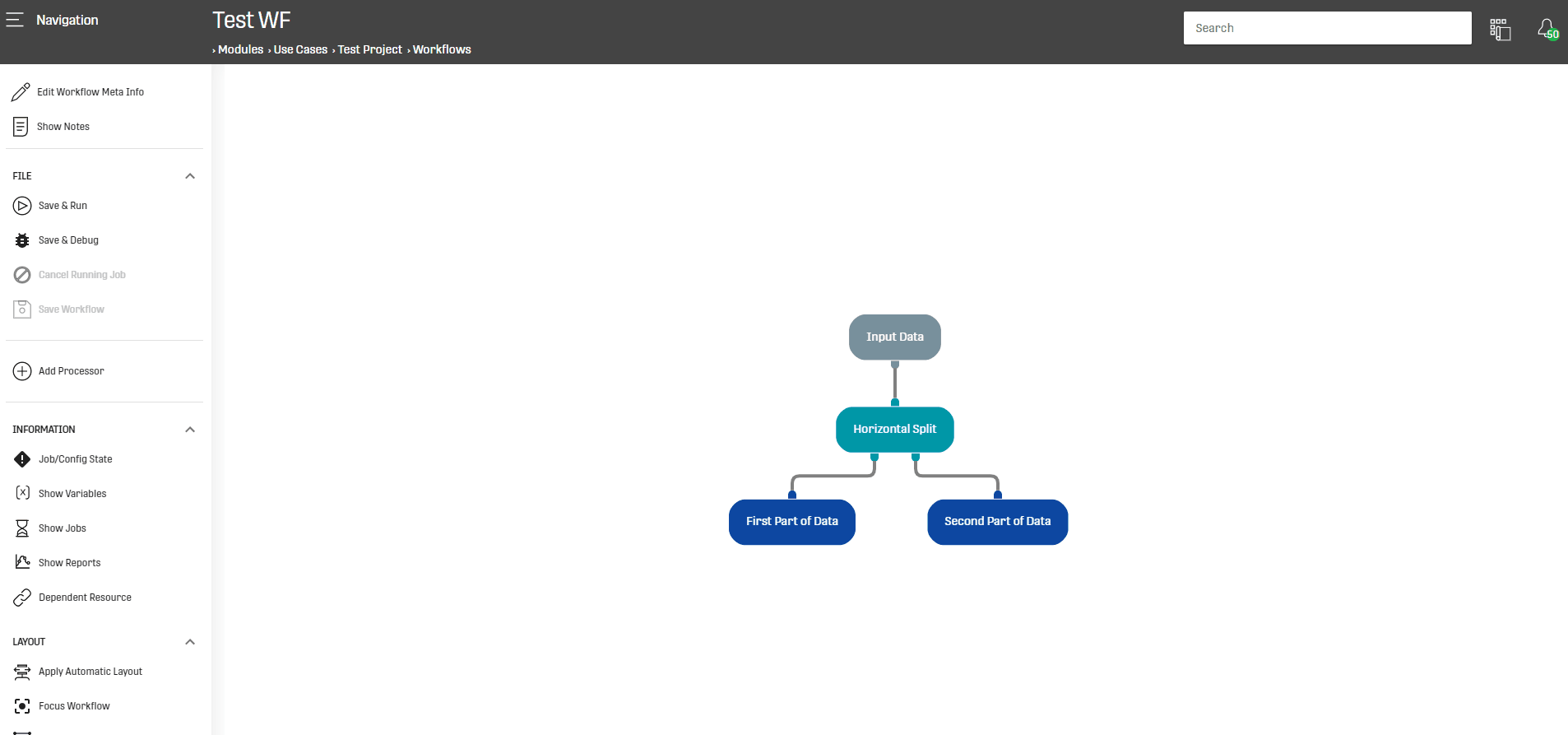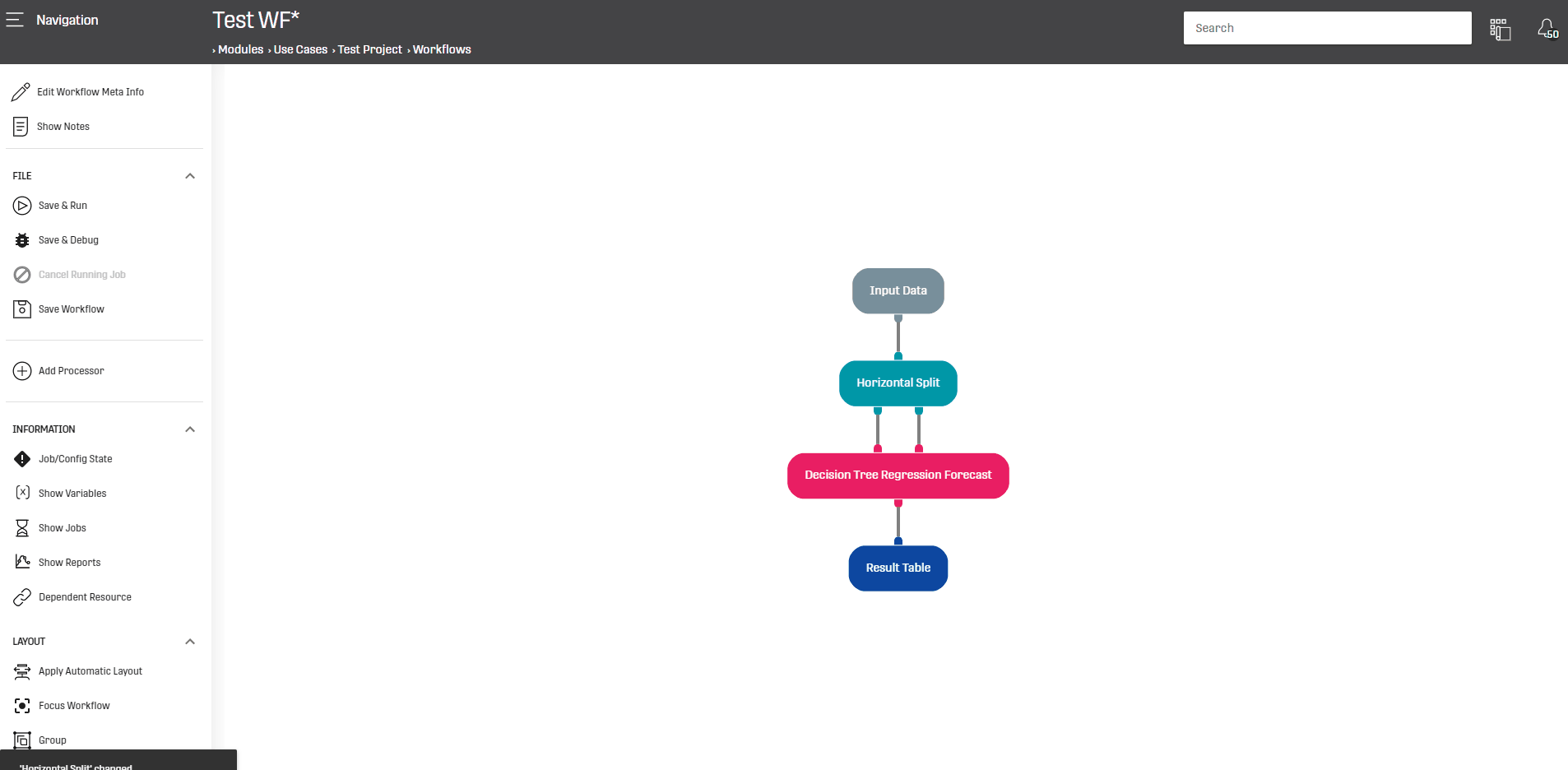# Overview

This processor is used to split the rows of a given Dataset.

This functionality is commonly used when training a model and it allows to divide the input Data into training and test Dataset.

# Configuration

This processor can operate on any valid Dataset, it can be configured as follows:NOTE THAT:
• If Ordering Column is specified (first field) then "seed" will be ignored as well as random splitting (last field)
• If no Ordering then "seed" will guarantee having the same output when running the workflow several times
• If no Ordering and no "seed" then splitting will be randomly done
• If no Ordering is specified then enabling the Random Split may severely impact performance

# Output

The processor has two output nodes:

• Left node: Contains the subset defined by the percentage value (third configuration field)
• Right node: Contains the remaining rows from the input Dataset.

The splitting operation is done randomly: meaning that it is not necessary that the first entries will be assigned to the left node.This leads to low bias of the model performance (and better coverage of data samples).

# Example

Here the Horizontal Split processor will be used to split a toy Dataset:Furthermore the Horizontal Split processor can be used to split Data that will be fed into a Machine Learning model such as Decision Tree Regression Forecast, Decision Tree Classification Forecast or even Train Model processor.

In the following example our processor of interest will feed Decision Tree Regression Forecast with training and test Data: